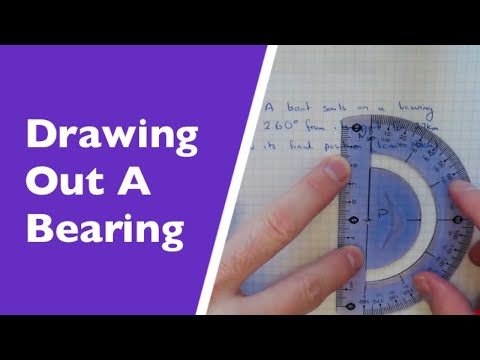## Scale Drawing and Bearing

Subject: Compulsory Maths

#### Overview

To draw the figure in the figure in the paper we can just reduce their actual size and for a small figure , we should enlarge their actual size in drawing by taking a scale . A scale drawing is defined as a drawing that shows the size of an object into the form which may be reduction or enlargement .The direction in which on object or place is sighted may be specified by giving the angle in degrees that the direction makes with the north line is called the bearing if the object. This note gives information about the bearing and scale drawing .

#### Scale Drawing :

As we know that we cannot draw the fixed shape and size of very small or very big figure on the sheet of paper . It is very difficult to show distances lengths or breath which are too big to draw full size . To draw the figure in the figure in the paper we can just reduce their actual size and for a small figure , we should enlarge their actual size in drawing by taking a scale . A scale drawing is defined as a drawing that shows the size of an object into the form which may be reduction or enlargement .

Scale Factor:

The scale of the drawing is the ratio of the size of the picture or figure to the same size of the objects. For example :

• A distance of 7Km.

Scale : 1cm to 1km ( or ,1:100000)Scale drawing .

The scale of a drawing is called scale factor . It is used to break (reduce) or increase (enlarge ) a figure according to the scale ratio.

Bearing:

You can see the adjoining compass and learn the different direction . Whereas in the compass NOS shows North-South and EOW show East - West direction . In the angle between N and E is 90o. Whereas the direction NE lies between N and E . The angle between N and NE is 45o. O is taken as the point of reference and ON as a base to find the direction of any place in degrees measuring clockwise from the baseline ( NO ) . The direction is usually written using three digits for example :

• The direction of NE from O is 045o. It is called bearing of NE from O.
• The direction of E from O is 090o. It is called bearing of E and O.
• Whereas the direction of SW from O is 225o. It is called bearing of SW from O and so on.

The direction in which one object or place is sighted may be specified by giving the angle in degrees that the direction makes with the north line is called the bearing if the object. Usually, the bearing of an object or place is written in three digits . So , it is also called three digit bearing.

##### Things to remember
• A scale drawing is defined as a drawing that shows the size of an object into the form which may be reduction or enlargement .
• The direction in which on object or place is sighted may be specified by giving the angle in degrees that the direction makes with the north line is called the bearing if the object.
• Bearing help shows the direction of the place .
• It includes every relationship which established among the people.
• There can be more than one community in a society. Community smaller than society.
• It is a network of social relationships which cannot see or touched.
• common interests and common objectives are not necessary for society.
##### Videos for Scale Drawing and Bearing##### Constructing Bearings. How To Draw A Bearing On A Map (Boat Problems)

Solution:

Here, 1 cm represents 5 m.

$\therefore$ 2.5 cm represents 2.5 × 5 m = 12.5 m ans.

Solution:

Here, the scale 1:500000 means, 1 cm represents 500000 cm = $\frac{500000}{100}$ m = 5000 m = 5 km

Now, 1 cm represents 5 km

$\therefore$ 3.2 represents 3.2 × 5 km = 16 km

So, the distance between two places is 16 km.

Solution:

Here, the scale 1:500 means, 1 cm represents 500 cm = 5 cm

5 m is represented by 1 cm.

1 m is represented by $\frac{1}{5}$.

25 m is represented by $\frac{1}{5}$ × 25 cm =5 cm

$\therefore$ The length of the room on drawing is 5 cm.

Also, 5 m is represented by 1 cm

1 m is represented by $\frac{1}{5}$ cm

18 m is represented by $\frac{1}{5}$ × 18 cm = 3.6 cm

$\therefore$ The breadth of the room on drawing is 3.6 cm.

Solution:

Here, bearing of A from B is 075°

Since, BN//AN, $\angle$NBA and $\angle$N1AB are co-interior angles.

$\therefore$ $\angle$ N1AB = 180° - 75° [Co-interior angles are supplementary.]

= 105°

$\therefore$ Bearing of B from A = 360° - 105° = 255°.